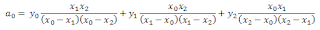### Secret Sharing Algorithm for Protecting Files in the Cloud

Data stored in the cloud can be compromised or lost (see my previous post). So, we have to come up with a way to secure those files. We can encrypt them before storing them in the cloud, which sorts out the disclosure aspects. However, what if the data is lost due to some catastrophe befalling the cloud service provider? We could store it on more than one cloud service and encrypt it before we send it off. Each of them will have the same file. What if we use an insecure, easily guessable password to protect the file, or the same one to protect all files? I have often thought that secret sharing algorithms could be employed to good effect in these circumstances instead.

What are secret sharing algorithms? They are algorithms that will share a secret between several parties, such that none of them can know the secret without the help of others. Either all or a subset of them will need to get together and put their parts together to obtain the original secret. A simplistic solution can be achieved by XORing the secret with a random number, then giving the result to one party and the random number to the other. Neither one can find out what the secret was without the other. To retrieve the secret they only need to XOR the two parts together again. This can be extended to any number of parties.

A more sophisticated way would be to allow the secret to be retrieved from a subset of the parts distributed. In the previous example, if any of the parties loses their part, or refuses to disclose it, then nobody can reveal the secret. This isn't much good if one of our cloud service providers fails. On the other hand, if we can share the secret between three people, but only require any two to regenerate the original, then we have some redundancy. This is an example of a (k,n) threshold scheme with k=2 and n=3.

How do we achieve this though? Well, Adi Shamir proposed a simple secure secret sharing algorithm. It is based on drawing graphs. To uniquely define a straight line, you need two points on that line. Similarly, to define a parabola you need three points. A cubic requires four, etc. So, we can distribute points on a line to each party we want to share the secret with. The order of the line will determine how many of them need to get together to regenerate it. So, we could define a random straight line and distribute three points on it to three different parties. However, only two of them need to get together to regenerate the original secret.

We set up a (k,n) threshold scheme by setting the free coefficient to be the secret and then choosing random numbers for each of the other coefficients. The polynomial then becomes the following:where a0 is our secret. Now we can distribute points on the line to each of the n parties simply by calculating y for a series of different values for x. We can use the Lagrange Basis Polynomials to reconstruct the equation of the line from k points. However, we do not need to reconstruct the whole line, we are only interested in the free term. This simplifies the equations that we need to use. For example, if we have a straight line, then we only need two points (x0,y0) and (x1,y1). We can then calculate a0 as follows:Similarly, for a parabola and three points (x0,y0), (x1,y1) and (x2,y2) we have:This should be fairly simple to implement and use. You would need to sign up to a few cloud services, but you wouldn't have all your eggs in one basket and you wouldn't be reliant on weak passwords.

1.Thanks for the secret secure algorithms for protecting files in the cloud. I think a secure secret sharing system is really helpful to allocate shares so that everyone shares with no extra information about the secret.